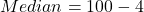Question

2071 Old Q.No.5 Person’s coefficient of skewness for a distribution is 0.4 and its coefficient of variation is 30%. If mode is 88, find mean and median.​

1.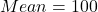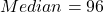Step-by-step explanation:

Given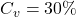— coefficient of variation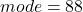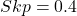Required

The mean and the median

The coefficient of variation is calculated using: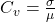Where: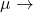mean

So: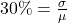Express percentage as decimal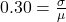Make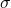the subject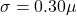The coefficient of skewness is calculated using: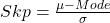This gives: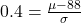Makethe subject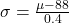Equate both expressions for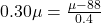Cross multiply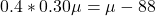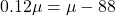Collect like terms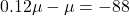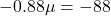Divide both sides by -0.88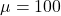Hence:Calculate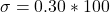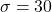So:

Also, the coefficient of skewness is calculated using: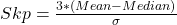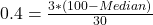Multiply both sides by 30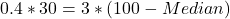Divide both sides by 3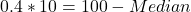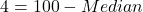Collect like terms